# Selina Solutions Concise Mathematics Class 6 Chapter 25 Properties of Angles and Lines Exercise 25(A)

Selina Solutions Concise Mathematics Class 6 Chapter 25 Properties of Angles and Lines Exercise 25(A) are designed by experts after conducting research on each concept. The sum of two adjacent angles in a straight line is 1800, which is the main concept discussed under this exercise. Students can improve problem solving and time management skills by using these solutions. This also helps them to appear for the examination fearlessly. To get their doubts cleared about the concepts, students can refer to Selina Solutions Concise Mathematics Class 6 Chapter 25 Properties of Angles and Lines Exercise 25(A) PDF, from the links provided here for free download.

## Selina Solutions Concise Mathematics Class 6 Chapter 25: Properties of Angles and Lines Exercise 25(A) Download PDF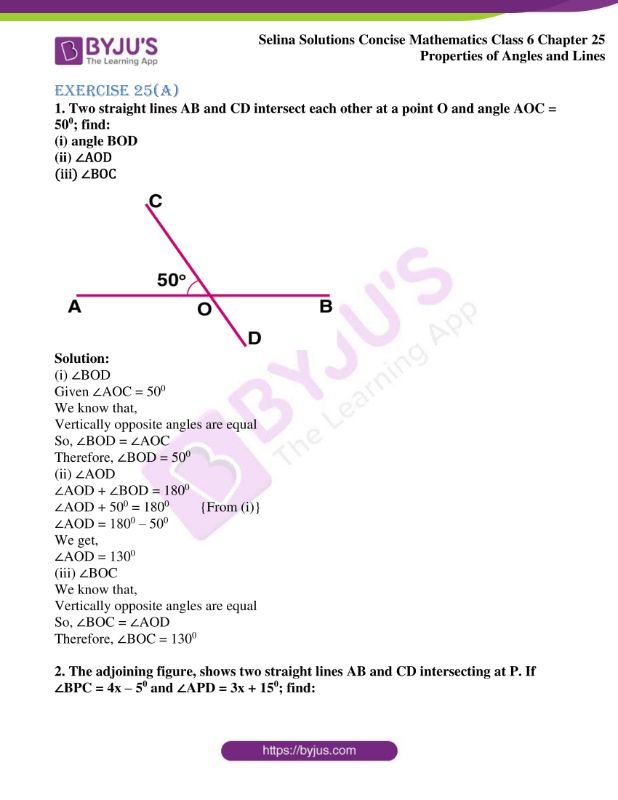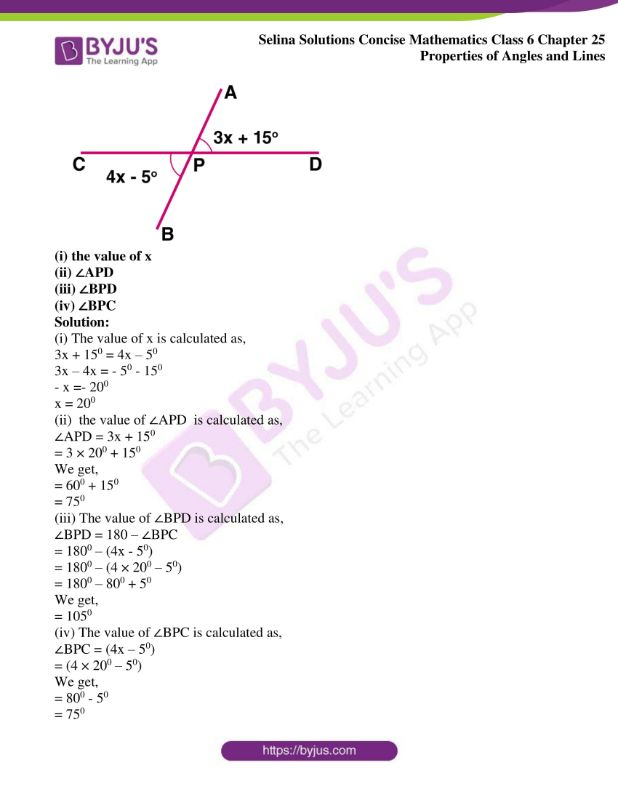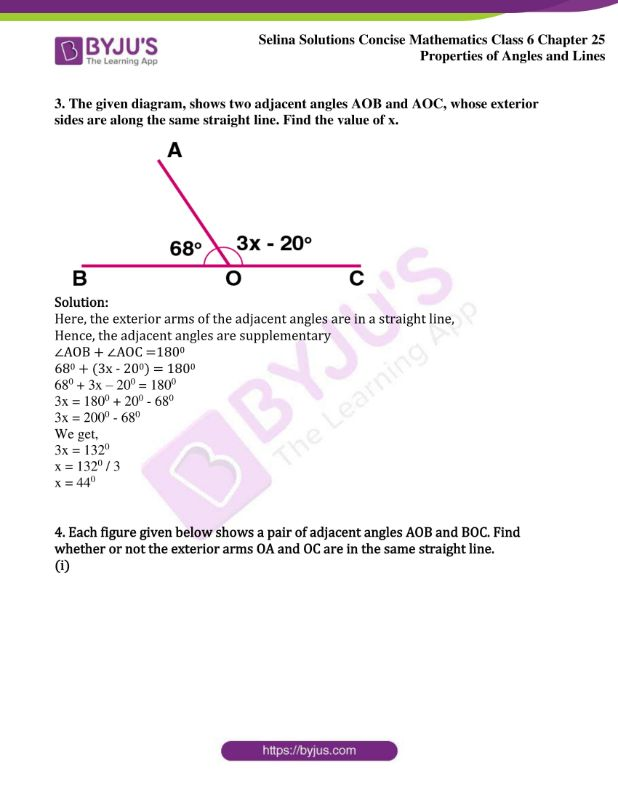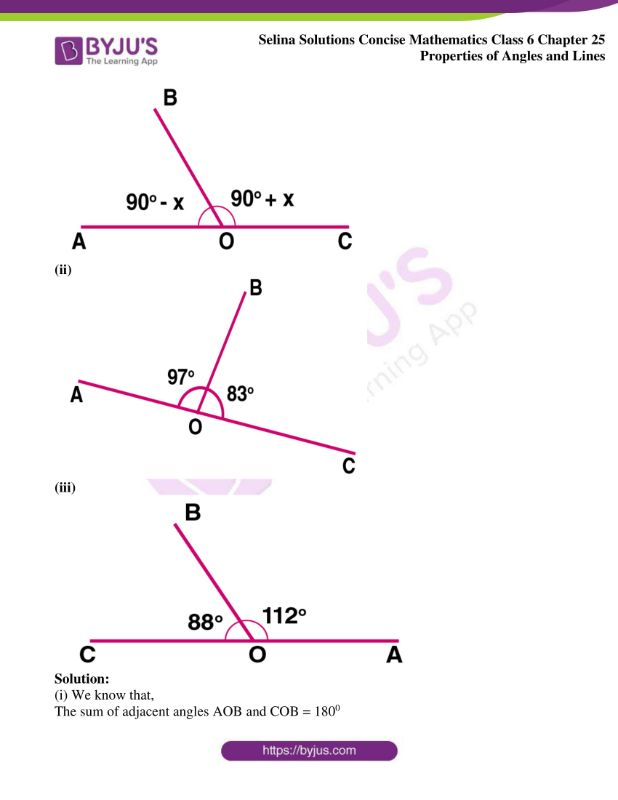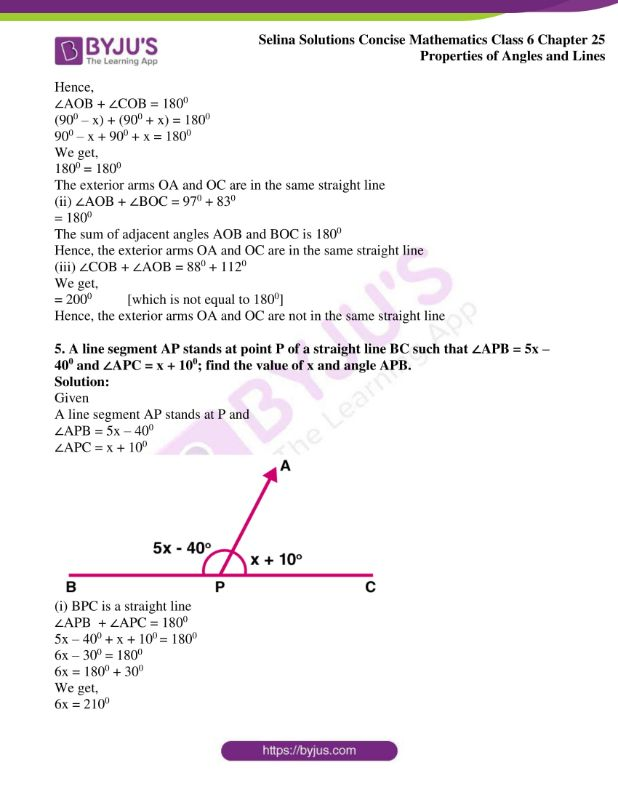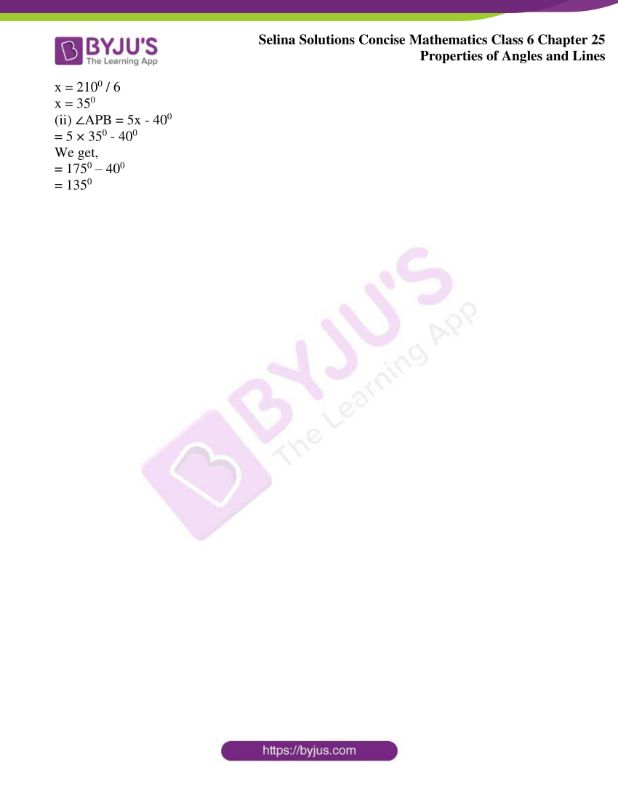### Access Selina Solutions Concise Mathematics Class 6 Chapter 25: Properties of Angles and Lines Exercise 25(A)

Exercise 25(A)

1. Two straight lines AB and CD intersect each other at a point O and angle AOC = 500; find:

(i) angle BOD

(ii) ∠AOD

(iii) ∠BOC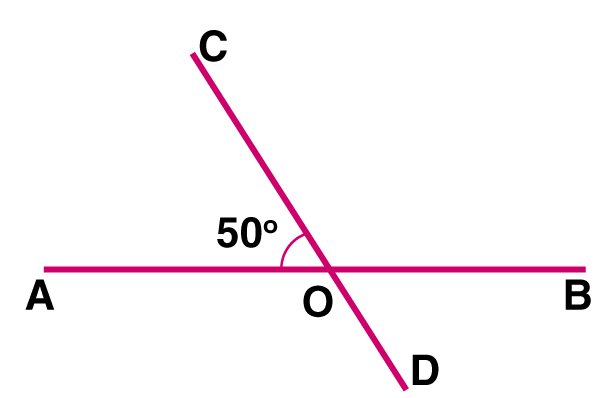Solution:

(i) ∠BOD

Given ∠AOC = 500

We know that,

Vertically opposite angles are equal

So, ∠BOD = ∠AOC

Therefore, ∠BOD = 500

(ii) ∠AOD

∠AOD + ∠BOD = 1800

∠AOD + 500 = 1800 {From (i)}

∠AOD = 1800 – 500

We get,

∠AOD = 1300

(iii) ∠BOC

We know that,

Vertically opposite angles are equal

So, ∠BOC = ∠AOD

Therefore, ∠BOC = 1300

2. The adjoining figure, shows two straight lines AB and CD intersecting at P. If ∠BPC = 4x – 50 and ∠APD = 3x + 150; find: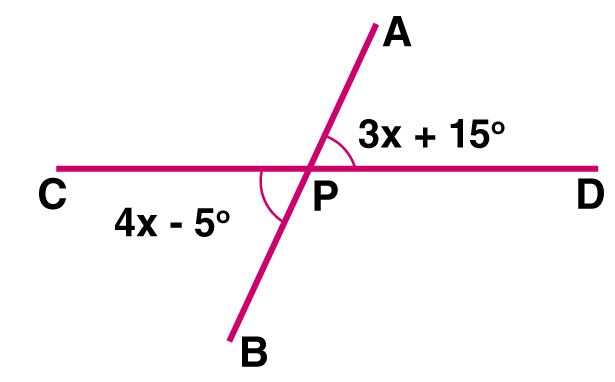(i) the value of x

(ii) ∠APD

(iii) ∠BPD

(iv) ∠BPC

Solution:

(i) The value of x is calculated as,

3x + 150 = 4x – 50

3x – 4x = – 50 – 150

– x =- 200

x = 200

(ii) the value of ∠APD is calculated as,

∠APD = 3x + 150

= 3 × 200 + 150

We get,

= 600 + 150

= 750

(iii) The value of ∠BPD is calculated as,

∠BPD = 180 – ∠BPC

= 1800 – (4x – 50)

= 1800 – (4 × 200 – 50)

= 1800 – 800 + 50

We get,

= 1050

(iv) The value of ∠BPC is calculated as,

∠BPC = (4x – 50)

= (4 × 200 – 50)

We get,

= 800 – 50

= 750

3. The given diagram, shows two adjacent angles AOB and AOC, whose exterior sides are along the same straight line. Find the value of x.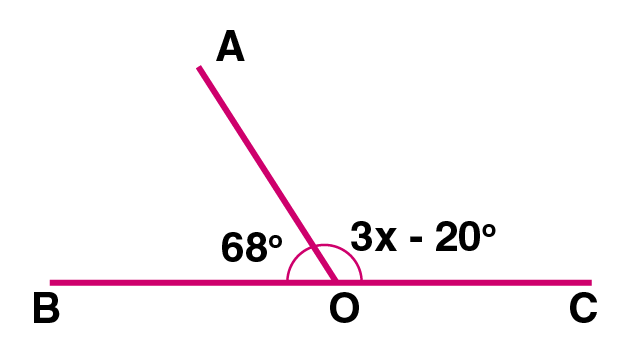Solution:

Here, the exterior arms of the adjacent angles are in a straight line,

Hence, the adjacent angles are supplementary

∠AOB + ∠AOC =1800

680 + (3x – 200) = 1800

680 + 3x – 200 = 1800

3x = 1800 + 200 – 680

3x = 2000 – 680

We get,

3x = 1320

x = 1320 / 3

x = 440

4. Each figure given below shows a pair of adjacent angles AOB and BOC. Find whether or not the exterior arms OA and OC are in the same straight line.

(i)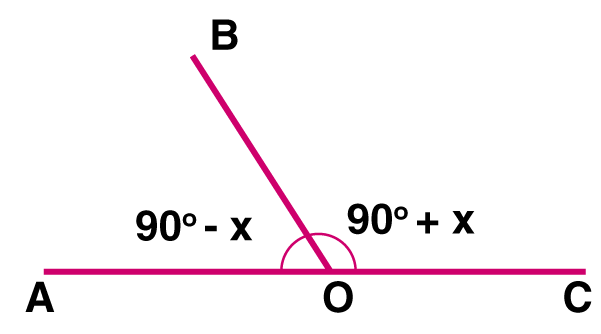(ii)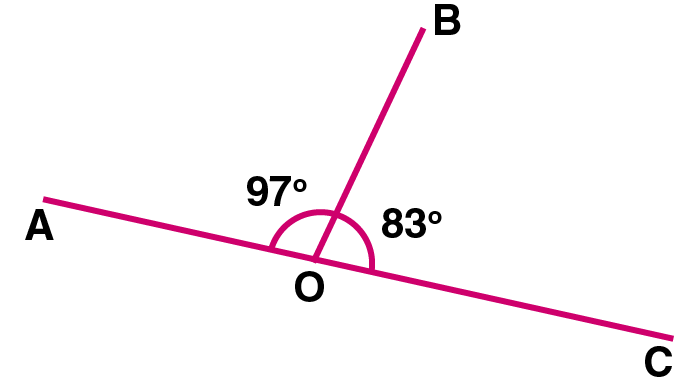(iii)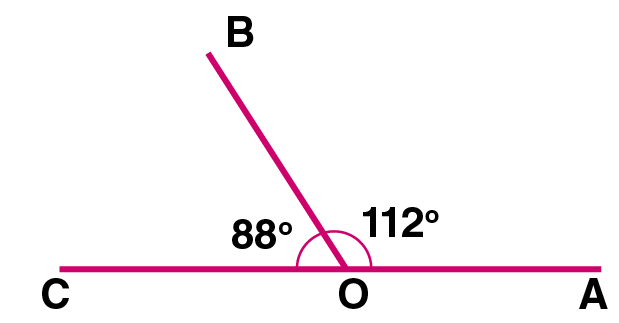Solution:

(i) We know that,

The sum of adjacent angles AOB and COB = 1800

Hence,

∠AOB + ∠COB = 1800

(900 – x) + (900 + x) = 1800

900 – x + 900 + x = 1800

We get,

1800 = 1800

The exterior arms OA and OC are in the same straight line

(ii) ∠AOB + ∠BOC = 970 + 830

= 1800

The sum of adjacent angles AOB and BOC is 1800

Hence, the exterior arms OA and OC are in the same straight line

(iii) ∠COB + ∠AOB = 880 + 1120

We get,

= 2000 [which is not equal to 1800]

Hence, the exterior arms OA and OC are not in the same straight line

5. A line segment AP stands at point P of a straight line BC such that ∠APB = 5x – 400 and ∠APC = x + 100; find the value of x and angle APB.

Solution:

Given

A line segment AP stands at P and

∠APB = 5x – 400

∠APC = x + 100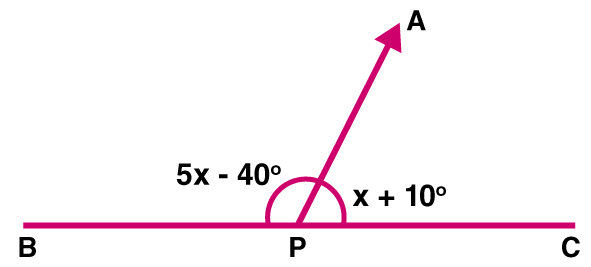(i) BPC is a straight line

∠APB + ∠APC = 1800

5x – 400 + x + 100 = 1800

6x – 300 = 1800

6x = 1800 + 300

We get,

6x = 2100

x = 2100 / 6

x = 350

(ii) ∠APB = 5x – 400

= 5 × 350 – 400

We get,

= 1750 – 400

= 1350